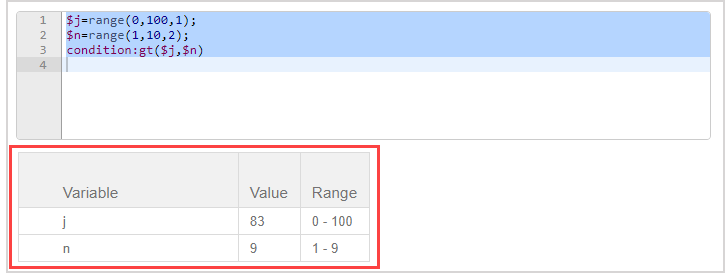# Create question algorithms using the Algorithm Designer

The Algorithm Designer (accessed from within the Algorithm Editor) helps you to build algorithmic commands without directly using algorithmic syntax.

TIP: Click Show Designer to reveal the Algorithm Designer entry fields.Click Hide Designer to collapse the Algorithm Designer.The Show Designer and Hide Designer buttons function the same in the Lesson or Assignment Editors, but are located below the Algorithm Editor.The Algorithm Designer has 4 sections that you can complete to build your algorithmic commands:• Random Number — Generates random values using range-based variables.
• Conditional Assignment — Generates explicit values based on defined relationships with other variables.
• Maple Command — Use Maple commands to define random values using Maple functions.
• Condition — Set rules that must be met when Möbius generates random values for variables.

You can complete as many sections as needed, and complete multiple versions of a single section to create multiple variables as needed. Example — Complete 3 iterations of the Random Number section to create 3 different variables.

TIP: Command lines can be removed by highlighting the desired line and pressing Delete on your keyboard.

TIP: You can manually edit the syntax of a command line in the Algorithm Editor even if the command was initially added using the Algorithm Designer.

NOTE: Variables must first be defined for an algorithmic question to display randomly-generated values based on the commands.

## Random Number section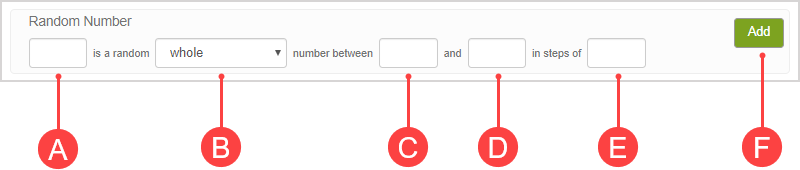1. (A) Enter the name of the variable. Examplen

1. (B) Select the type of number to be generated from the drop-down list. Examplewhole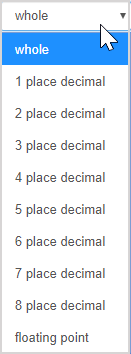1.  (C) Enter the lower bound of the numerical range. Example1

1.  (D) Enter the upper bound of the numerical range. Example10

1. (E) Enter the increment to be used. Example2

1. (F) Click Add to translate these parameters into algorithmic syntax.

1. The algorithmic command is translated to algorithmic syntax and is visible in the Algorithmic Editor.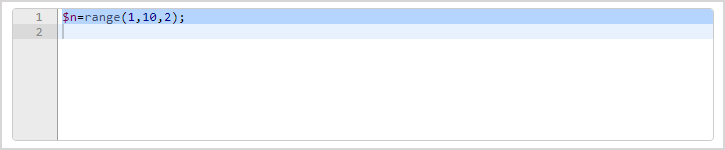TIP: Click Refresh algorithm preview (or Refresh if using the Algorithm Designer in the Lesson or Assignment Editors) as many times as needed to see examples of what values would be generated based on your new algorithmic command.Variations of the variable(s) are displayed using your algorithmic command.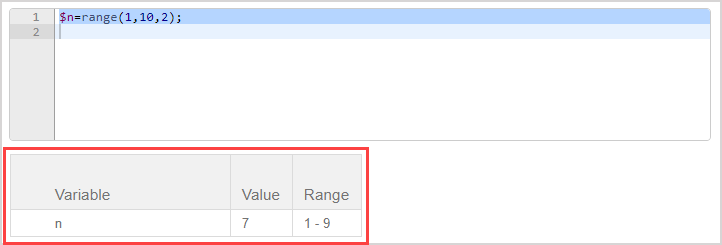## Conditional Assignment section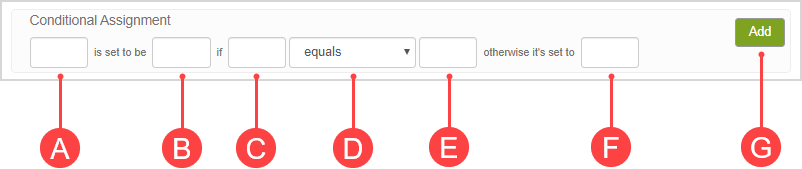NOTE: Any variables referenced within the condition statement must already be defined by previous commands.

1. (A) Enter the name of the variable that will be affected if the condition is satisfied. Examplen

1. (B) Enter the value that the affected variable will be if the condition is satisfied. Example3

1. (C) Enter the name of a second variable that the first variable is dependent on for the condition. Examplej

1. (D) Selecting the relation from the drop-down list. Exampleequals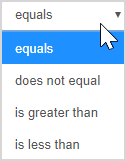1. (E) Enter the value that the second variable must be to satisfy the condition. Example50

1. (F) Enter the value that the first variable will be if the condition isn't satisfied. Example9

1. (G) Click Add to translate these parameters into algorithmic syntax.

1. The algorithmic command is translated to algorithmic syntax and is visible in the Algorithmic Editor.TIP: Click Refresh algorithm preview (or Refresh if using the Algorithm Designer in the Lesson or Assignment Editors) as many times as needed to see examples of what values would be generated based on your new algorithmic command.Variations of the variable(s) are displayed using your algorithmic command.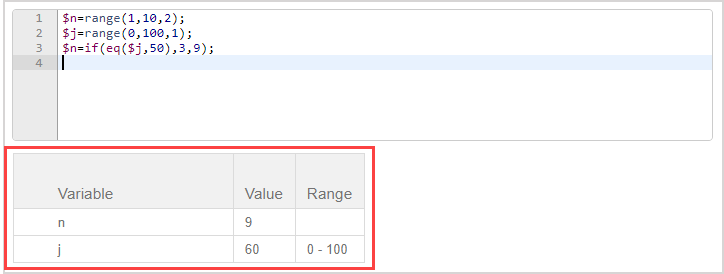## Maple Command section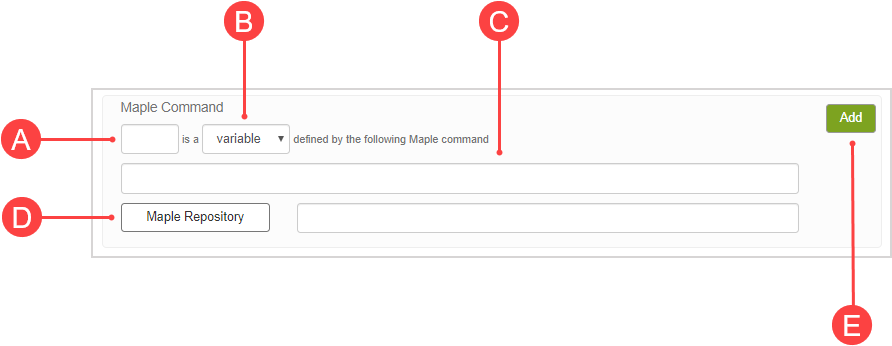1. (A) Enter the name of the variable. Exampleu

1. (B) Select the type of variable to generate from the drop-down list. Examplevariable1. (C) Enter the Maple commands used to define the random variable or enter the plot statement. The plot statement accepts plot device options. Examplediff(x^\$n+cos(x), x);

NOTE: Rules for Maple commands:

• Use multiple commands: randomize() : <random_command> for each random variable (otherwise, the same output is generated in each instantiation of the question).
• Ensure that all commands have a trailing semi-colon (;) when entering multiple commands.
• Use the \$ or \${ } notation when including variables in a Maple command.
• Place the negative variable in parentheses when referencing negative random variables in a Maple variable definition (otherwise an error message will appear).
1.  (Optional) You can also link to a Maple repository when generating random variables if you've created custom Maple commands. (D) Click Maple Repository to import and link to a Maple repository from the Class File Manager to reference your commands.

1. (E) Click Add to translate these parameters into algorithmic syntax including Maple code.

1. The algorithmic command is translated to algorithmic syntax including Maple code and is visible in the Algorithmic Editor.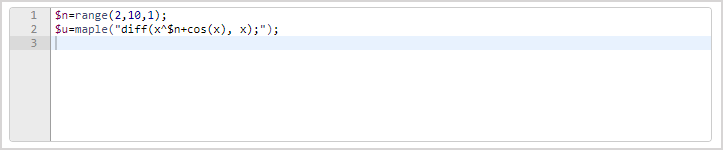TIP: Click Refresh algorithm preview (or Refresh if using the Algorithm Designer in the Lesson or Assignment Editors) as many times as needed to see examples of what values would be generated based on your new algorithmic command.Variations of the variable(s) are displayed using your algorithmic command.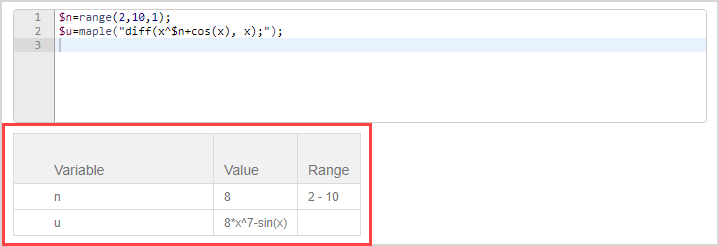## Condition section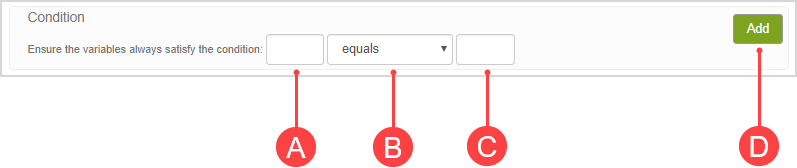NOTE: Any variables referenced within the condition statement must already be defined by previous commands.

1. (A) Enter the name of a variable that's to be affected by the condition. Examplej

1. (B) Select the conditional scenario from the drop-down list. Exampleis greater than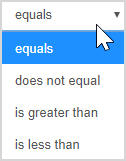1. (C) Enter the name of a second variable that is affected by the condition or a specific value. Examplen

1. (D) Click Add to translate these parameters into algorithmic syntax.

1. The algorithmic command is translated to algorithmic syntax and is visible in the Algorithmic Editor.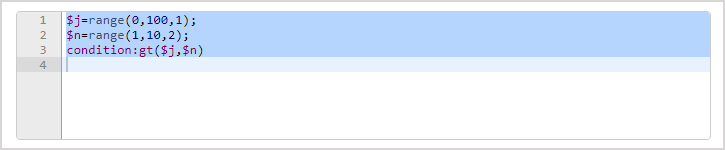TIP: Click Refresh algorithm preview (or Refresh if using the Algorithm Designer in the Lesson or Assignment Editors) as many times as needed to see examples of what values would be generated based on your new algorithmic command.Variations of the variable(s) are displayed using your algorithmic command.